#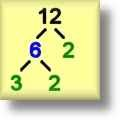Factors Worksheets

## Printable Factors and Multiples Worksheets

Here is a graphic preview for all of the Factors Worksheets. You can select different variables to customize these Factors Worksheets for your needs. The Factors Worksheets are randomly created and will never repeat so you have an endless supply of quality Factors Worksheets to use in the classroom or at home. Our Factors Worksheets are free to download, easy to use, and very flexible.

## Quick Link for All Factors Worksheets

Click the image to be taken to that Factors Worksheet.

##### Prime Factorization TreesFactors Worksheets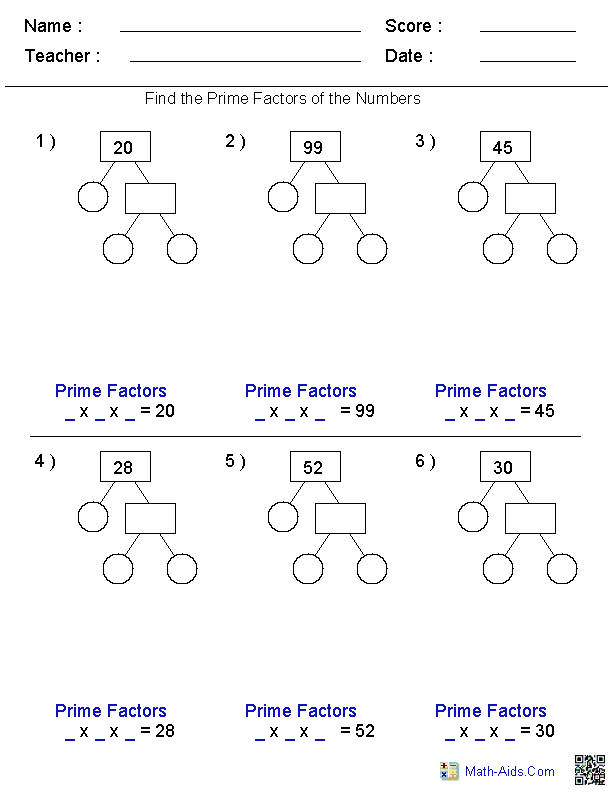##### Greatest Common FactorFactors Worksheets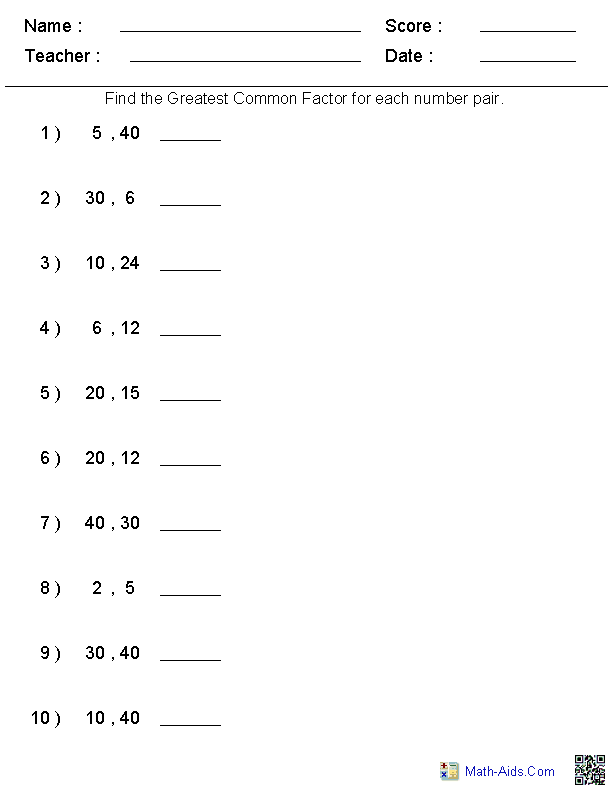##### Least Common MultipleFactors Worksheets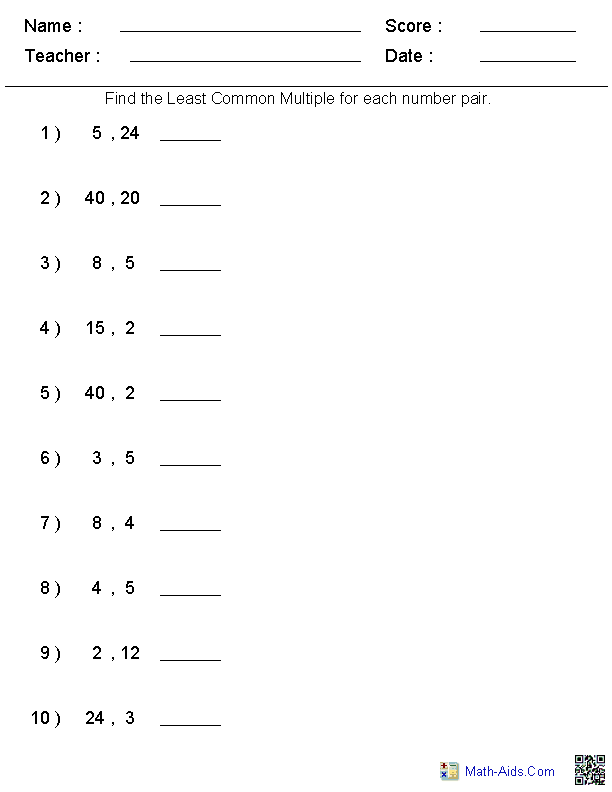##### Least Common MultipleGreatest Common Factor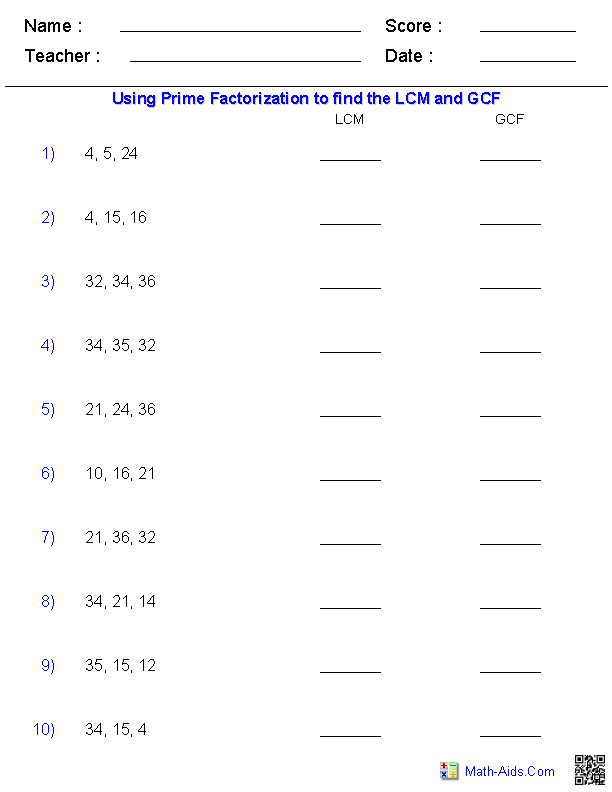##### List All FactorsFactors Worksheets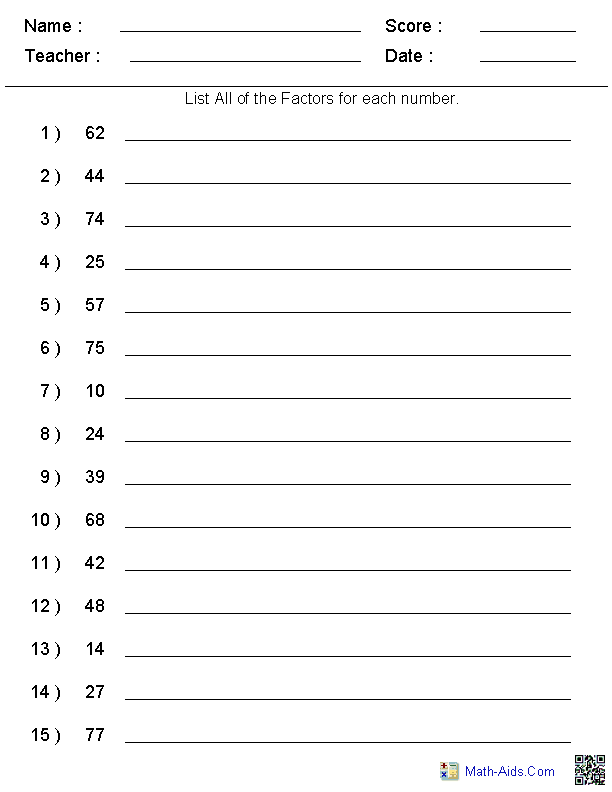##### List Prime FactorsFactors Worksheets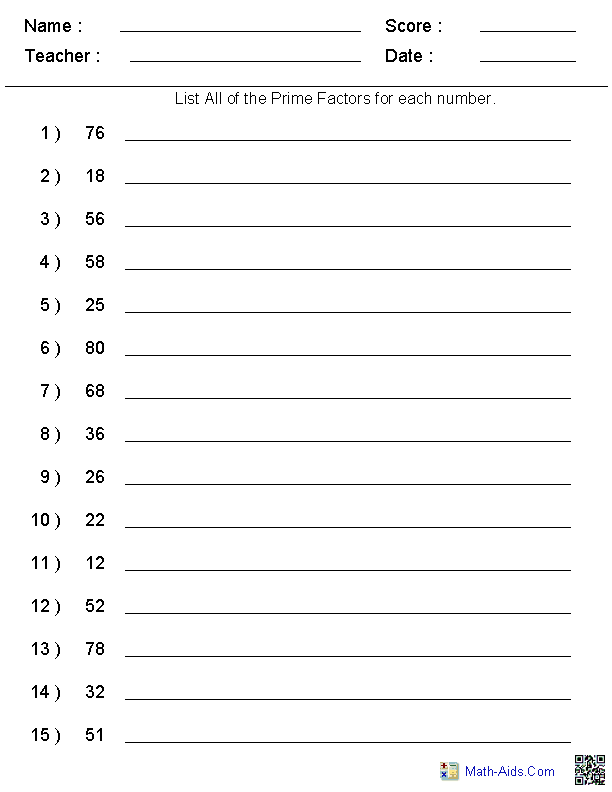##### List Prime FactorizationFactors Worksheets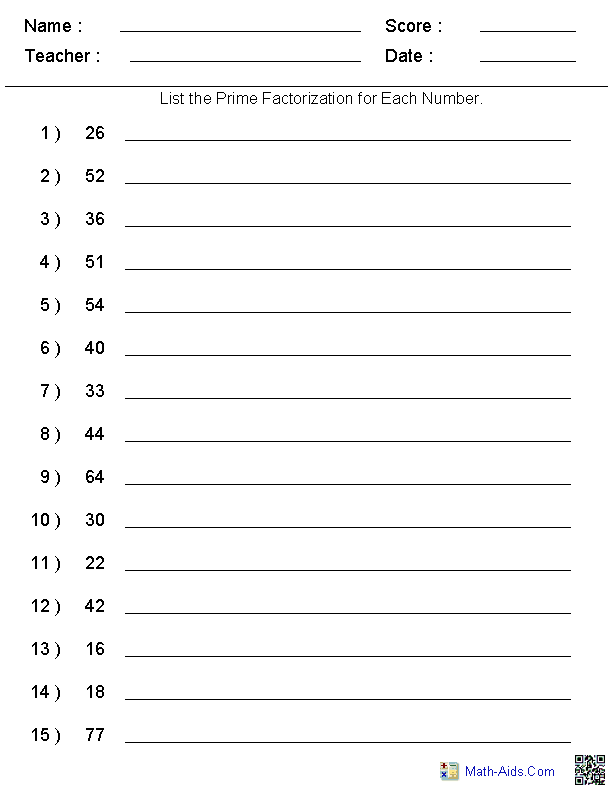Recommended Videos

## Detailed Description for All Factors Worksheets

Prime Factorization Trees Worksheets
These factor tree worksheets are great for practicing finding all of the prime factors contained in a number. The Prime Factorization Trees Worksheets are great visual aids. These factor tree worksheets will generate 6 Prime Factorization Tree problems per worksheet.

Greatest Common Factor Worksheets
These factors worksheets are great for practicing finding the Greatest Common Factor of number sets. These factors worksheets will generate 10, 15 or 20 Greatest Common Factor problems per worksheet.

Least Common Multiple Worksheets
These factors worksheets are great for practicing finding the Least Common Multiple of number sets. The problems may be selected from four different number ranges. These factors worksheets will generate 10, 15 or 20 Least Common Multiple problems per worksheet.

Least Common Multiple and Greatest Common Factor Worksheets
These factors worksheets are great for practicing finding the Least Common Multiple and Greatest Common Factor of number sets. The problems may be selected from four different number ranges. These factors worksheets will generate 10 or 20 problems per worksheet.

List All Factors Worksheets
These factors worksheets are great for practicing finding all of the Factors of number. These factors worksheets will generate 10, 15 or 20 Factor problems per worksheet.

List Prime Factors Worksheets
These factors worksheets are great for practicing finding the Prime Factors for a number. These factors worksheets will generate 10, 15 or 20 Prime Factor problems per worksheet.

List Prime Factorization Worksheets
These factors worksheets are great for practicing solving the Prime Factorization for a number. These factors worksheets will generate 10, 15 or 20 Prime Factorization problems per worksheet.